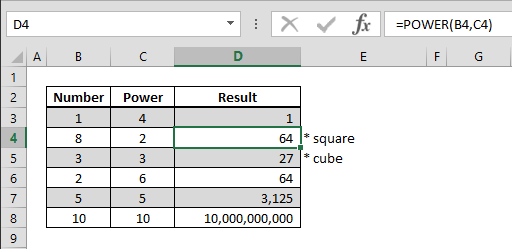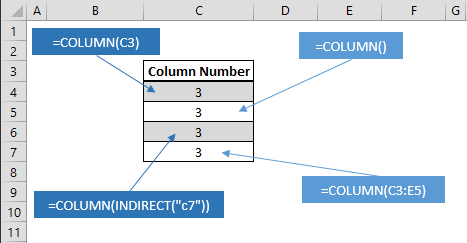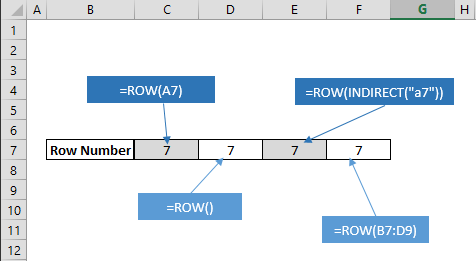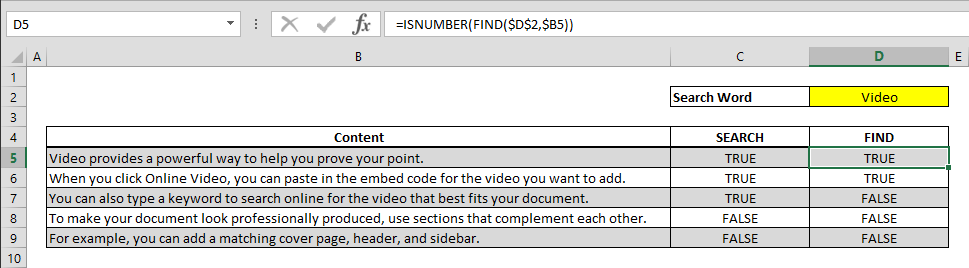## How to find the power of a number

You can always use caret symbol (^) to find a certain power of a number. However, doing this with a formula can be easier to read and identify in nested formula sets. Syntax =POWER(base number, how many times that base number will be multiplied by itself) Steps Begin...## How to get the column index of a cell using the COLUMN function

Working with Excel’s coordinate system makes building data models extremely easy. You can retrieve the column index information of a cell using the COLUMN function and implement in other formulas. Syntax =COLUMN(cell or range reference you want get its column...## How to get the row index of a cell using the ROW function

Working with Excel’s coordinate system makes building data models extremely easy. You can retrieve the row index information of a cell using the ROW function and implement in other formulas. Syntax =ROW(cell or range reference you want get its row number) Steps...## How to search for a string or check if cell contains a specific text

Excel’s Search and Find feature is very helpful when looking up things in workbooks, but this means searching for keywords one by one. SEARCH and FIND are formula versions of this very handy feature and can work wonders in large data sets. Syntax...## Handle With Care: Volatile Functions in Excel

You can create all sorts of mathematical models in Excel, but imagine a model with thousands of formulas, all of which need to be calculated every time you enter a value. This would make calculations very slow and the user experience, not a pleasant one. Thanks to its...## INDIRECT Formula and Proper Referencing

INDIRECT is a versatile function in Excel’s already huge repertoire of referencing tools. In this guide we’re going to take a look at its various use cases. This function can return the reference specified by a text string, foolproof your Excel models, or even...